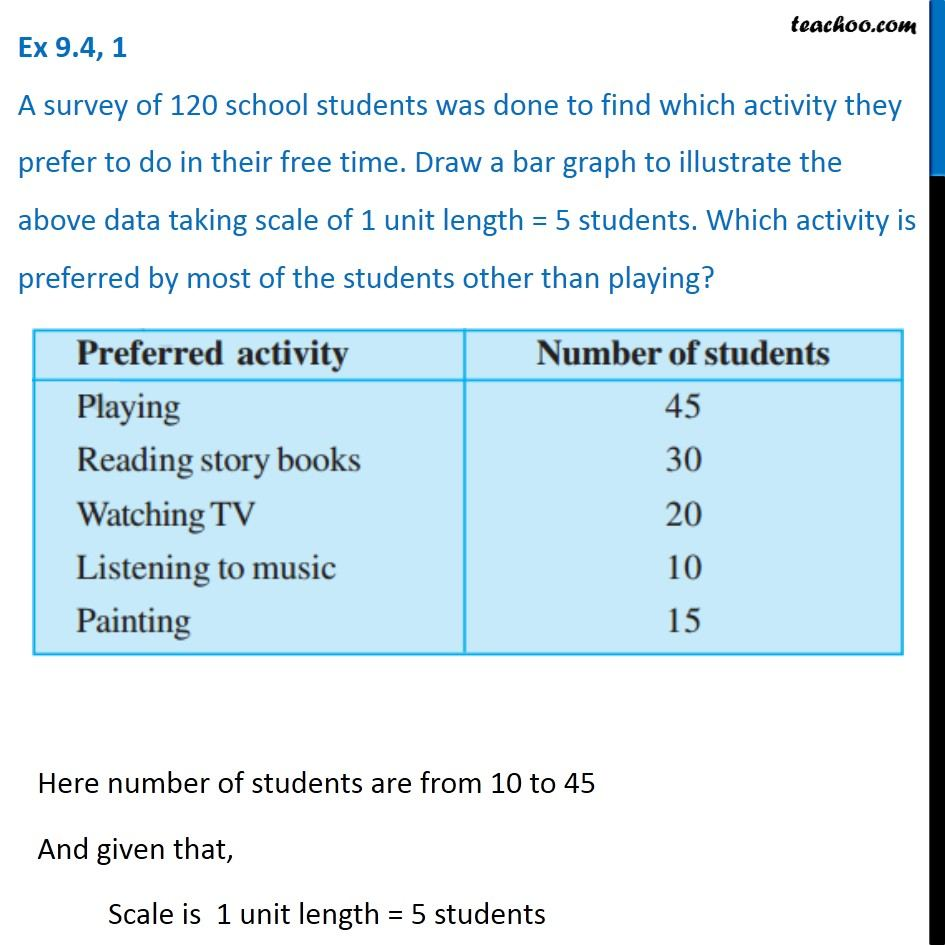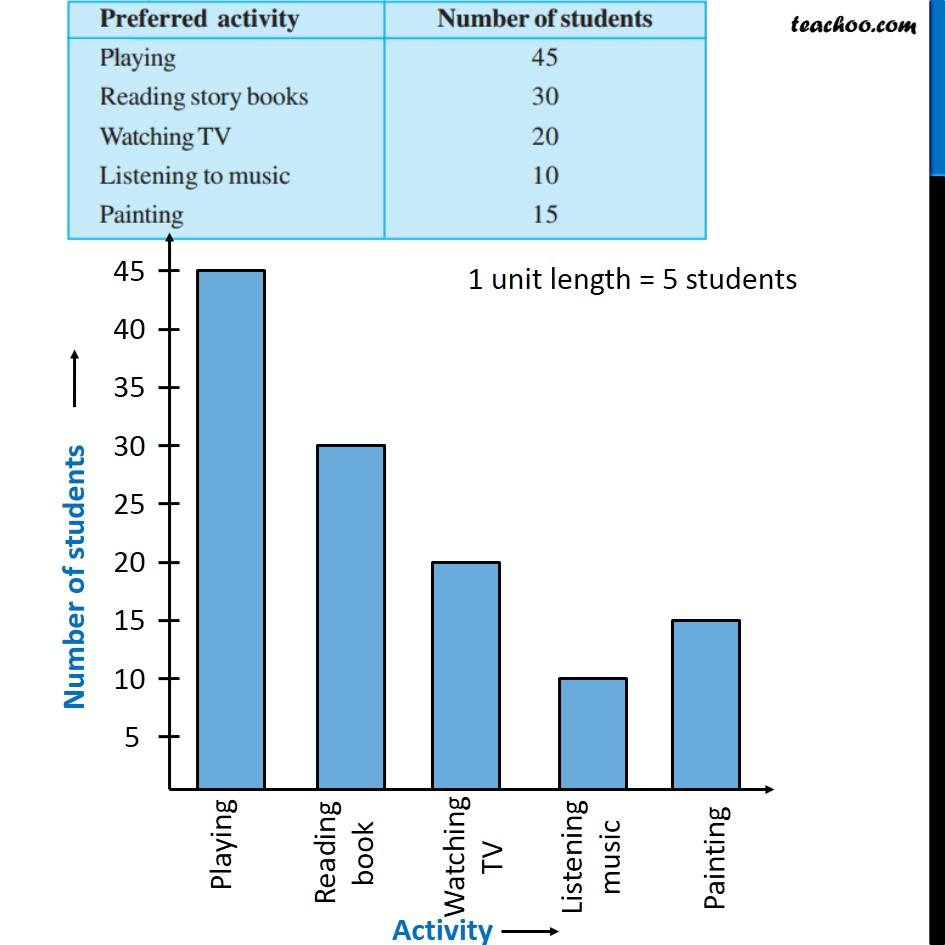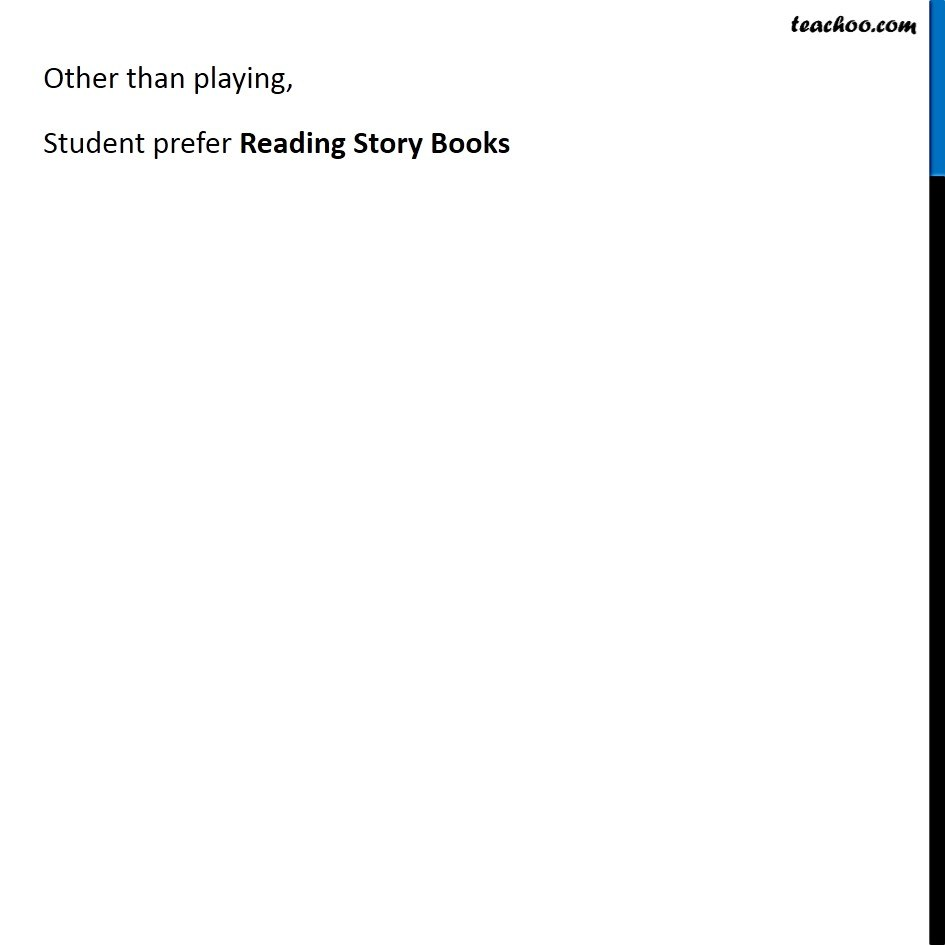1. Chapter 9 Class 6 Data Handling
2. Concept wise
3. Drawing bar graph

Transcript

Ex 9.4, 1 A survey of 120 school students was done to find which activity they prefer to do in their free time. Draw a bar graph to illustrate the above data taking scale of 1 unit length = 5 students. Which activity is preferred by most of the students other than playing? Here number of students are from 10 to 45 And given that, Scale is 1 unit length = 5 students Other than playing, Student prefer Reading Story Books

Drawing bar graph